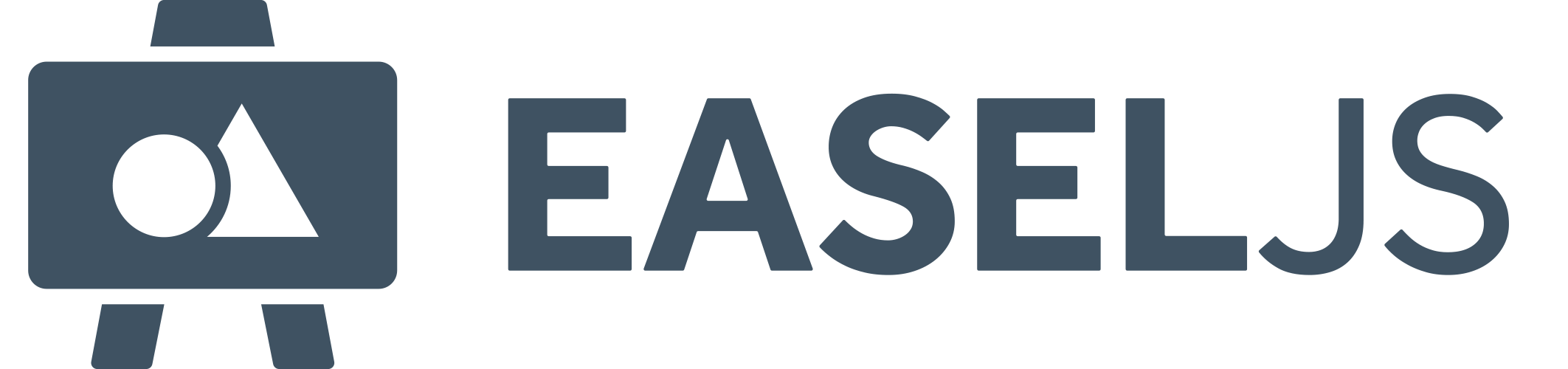#API Documentation for: 1.0.0
Show:

# ColorFilter Class

Extends Filter
Module: EaselJS

Applies a color transform to DisplayObjects.

#### Example

This example draws a red circle, and then transforms it to Blue. This is accomplished by multiplying all the channels to 0 (except alpha, which is set to 1), and then adding 255 to the blue channel.

`````` var shape = new createjs.Shape().set({x:100,y:100});
shape.graphics.beginFill("#ff0000").drawCircle(0,0,50);

shape.filters = [
new createjs.ColorFilter(0,0,0,1, 0,0,255,0)
];
shape.cache(-50, -50, 100, 100);``````

## Constructor

### `ColorFilter`

(
• `[redMultiplier=1]`
• `[greenMultiplier=1]`
• `[blueMultiplier=1]`
• `[alphaMultiplier=1]`
• `[redOffset=0]`
• `[greenOffset=0]`
• `[blueOffset=0]`
• `[alphaOffset=0]`
)

#### Parameters:

• `[redMultiplier=1]` Number optional

The amount to multiply against the red channel. This is a range between 0 and 1.

• `[greenMultiplier=1]` Number optional

The amount to multiply against the green channel. This is a range between 0 and 1.

• `[blueMultiplier=1]` Number optional

The amount to multiply against the blue channel. This is a range between 0 and 1.

• `[alphaMultiplier=1]` Number optional

The amount to multiply against the alpha channel. This is a range between 0 and 1.

• `[redOffset=0]` Number optional

The amount to add to the red channel after it has been multiplied. This is a range between -255 and 255.

• `[greenOffset=0]` Number optional

The amount to add to the green channel after it has been multiplied. This is a range between -255 and 255.

• `[blueOffset=0]` Number optional

The amount to add to the blue channel after it has been multiplied. This is a range between -255 and 255.

• `[alphaOffset=0]` Number optional

The amount to add to the alpha channel after it has been multiplied. This is a range between -255 and 255.

## Methods

### `_applyFilter`

(
• `imageData`
)

#### Parameters:

• `imageData` ImageData

Target ImageData instance.

### `applyFilter`

(
• `ctx`
• `x`
• `y`
• `width`
• `height`
• `[targetCtx]`
• `[targetX]`
• `[targetY]`
)

Applies the filter to the specified context.

#### Parameters:

• `ctx` CanvasRenderingContext2D

The 2D context to use as the source.

• `x` Number

The x position to use for the source rect.

• `y` Number

The y position to use for the source rect.

• `width` Number

The width to use for the source rect.

• `height` Number

The height to use for the source rect.

• `[targetCtx]` CanvasRenderingContext2D optional

The 2D context to draw the result to. Defaults to the context passed to ctx.

• `[targetX]` Number optional

The x position to draw the result to. Defaults to the value passed to x.

• `[targetY]` Number optional

The y position to draw the result to. Defaults to the value passed to y.

#### Returns:

Boolean:

If the filter was applied successfully.

### `clone`

()

Returns a clone of this Filter instance.

#### Returns:

Filter:

A clone of the current Filter instance.

### `getBounds`

(
• `[rect]`
)

Provides padding values for this filter. That is, how much the filter will extend the visual bounds of an object it is applied to.

#### Parameters:

• `[rect]` Rectangle optional

If specified, the provided Rectangle instance will be expanded by the padding amounts and returned.

#### Returns:

Rectangle:

If a `rect` param was provided, it is returned. If not, either a new rectangle with the padding values, or null if no padding is required for this filter.

### `shaderParamSetup`

(
• `gl`
• `stage`
• `shaderProgram`
)

Assign any unique uniforms or other setup functionality here.

#### Parameters:

• `gl` WebGLContext

The context associated with the stage performing the render.

• `stage` StageGL

The stage instance that will be rendering.

• `shaderProgram` ShaderProgram

The compiled shader that is going to be used to perform the render.

### `toString`

()

Returns a string representation of this object.

#### Returns:

String:

a string representation of the instance.

## Properties

### `_multiPass`

Filter private

Another filter that is required to act as part of this filter and created and managed under the hood.

Default: null

### `alphaMultiplier`

Number

Alpha channel multiplier.

### `alphaOffset`

Number

Alpha channel offset (added to value).

### `blueMultiplier`

Number

Blue channel multiplier.

### `blueOffset`

Number

Blue channel offset (added to value).

### `FRAG_SHADER`

Pre-processed template shader code. It will be parsed before being fed in into the shader compiler. This should be based upon StageGL.SHADER_FRAGMENT_BODY_REGULAR

### `greenMultiplier`

Number

Green channel multiplier.

### `greenOffset`

Number

Green channel offset (added to value).

### `redMultiplier`

Number

Red channel multiplier.

### `redOffset`

Number

Red channel offset (added to value).

### `usesContext`

Boolean

A flag stating that this filter uses a context draw mode and cannot be batched into imageData processing.

Default: false